# FPROPS/Viscosity

Calculation of viscosity in FPROPS is currently in development. Ultimately, different publications provide different types of correlations (equations), so we implement a system that permits alternative correlations to be selected.

## First correlation

Driven by the use-case of transport properties for supercritical carbon dioxide, the first required viscosity correlation follows this form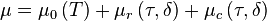$\mu = \mu_0 \left(T \right) + \mu_r \left(\tau,\delta \right) + \mu_c \left(\tau, \delta \right)$

where dynamic viscosity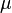$\mu$ is the sum of an ideal (zero-pressure) component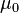$\mu_0$, a residual component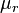$\mu_r$, and optionally, a critical-region component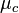$\mu_c$. Note that viscosity is said to increase to infinity in the vicinity of the critical point (which seems to suggest that it would make more sense to calculate in terms of something like an 'inviscidity' instead...?)

The ideal part is calculated as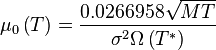$\mu_0 \left( T \right) = \frac{0.0266958 \sqrt{MT}}{\sigma^2 \Omega \left(T^{{}*{}}\right)}$

Here,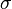$\sigma$ is referred to as the Lennard-Jones size parameter, and is a length value specific to each fluid.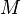$M$ is the molecular mass. The units of the value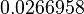$0.0266958$ need to be determined; Lemmon & Jacobsen state that the result as-written is returned in units of µPa·s. The expression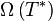$\Omega\left(T^{{}*{}}\right)$ is referred to as the collision integral and is a series with fluid-specific coefficients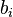$b_i$ and exponents$i$, calculated as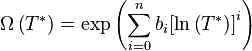$\Omega \left( T^{{}*{}} \right) = \exp \left(\sum_{i=0}^{n}{b_i {\left[\ln \left( T^{{}*{}} \right) \right]} ^i} \right)$

The residual part is calculated more simply, as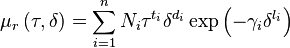$\mu_r \left(\tau,\delta\right) = \sum_{i=1}^{n}{N_i \tau^{t_i} \delta^{d_i} \exp \left(-\gamma_i \delta^{l_i} \right)}$

where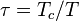$\tau = T_c / T$,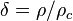$\delta = \rho / \rho_c$, and fluid-specific correlation data provides a table with n rows of values for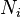$N_i$,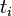$t_i$,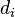$d_i$ and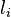$l_i$. The coefficient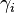$\gamma_i$ is defined as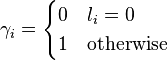$\gamma_i = \begin{cases} 0 & l_i = 0 \\ 1 & \mbox{otherwise} \end{cases}$

The critical-point component,$\mu_c$ is not yet implemented.